# Explanation of results: Powers and losses

## Introduction

It is necessary to give more details about the computation of different powers and losses.

## Active power and Joule losses: Why?

In the steady state AC application, Flux can compute:

• the apparent power S = ModC(PowV)
• the reactive power Q = Imag(PowV)
• the active power P = Real(PowV)
• the Joule losses:
• region of coil conductor type: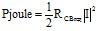• region of solid conductor type: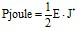Question: the active power and Joule losses are they identical?

Answer: yes and no, according to the modeled case. (see following block)

## Formula details

Here is a reminder of formulas in steady state AC application: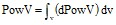with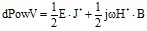The first part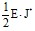is mandatory a real because J* can't be complex.

But the second part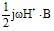can have a real part and an imaginary part when the case is modeled with a complex permeability:

• if ì isn't complex → active power = Joule losses
• si ì is complex → active power ≠ Joule losses

## Joule losses (coil conductor)

Two possibilities to compute Joule losses of a coil conductor:

• on a region: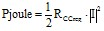(in steady state AC Magnetic)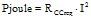(in transient magnetic)

with RCCreg = resistance computed in function of the material and of the fill factor* associated to the region of coil conductor coil*.

• on a component: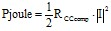(in steady state AC Magnetic)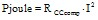(in transient magnetic)

with RCCcomp = RCCreg + RCCadd

where RCCadd corresponding to the resistance defined in the coil conductor

Note: * To compute RCCreg, it must mandatory that the corresponding region have an associated material (defined with a resistivity) AND a fill factor (between 0 and 1) which are optional fields.

If one field is missed, the computation on region of Joule losses is « Invalid » and the computation on component of Joule losses takes into account only the RCCadd